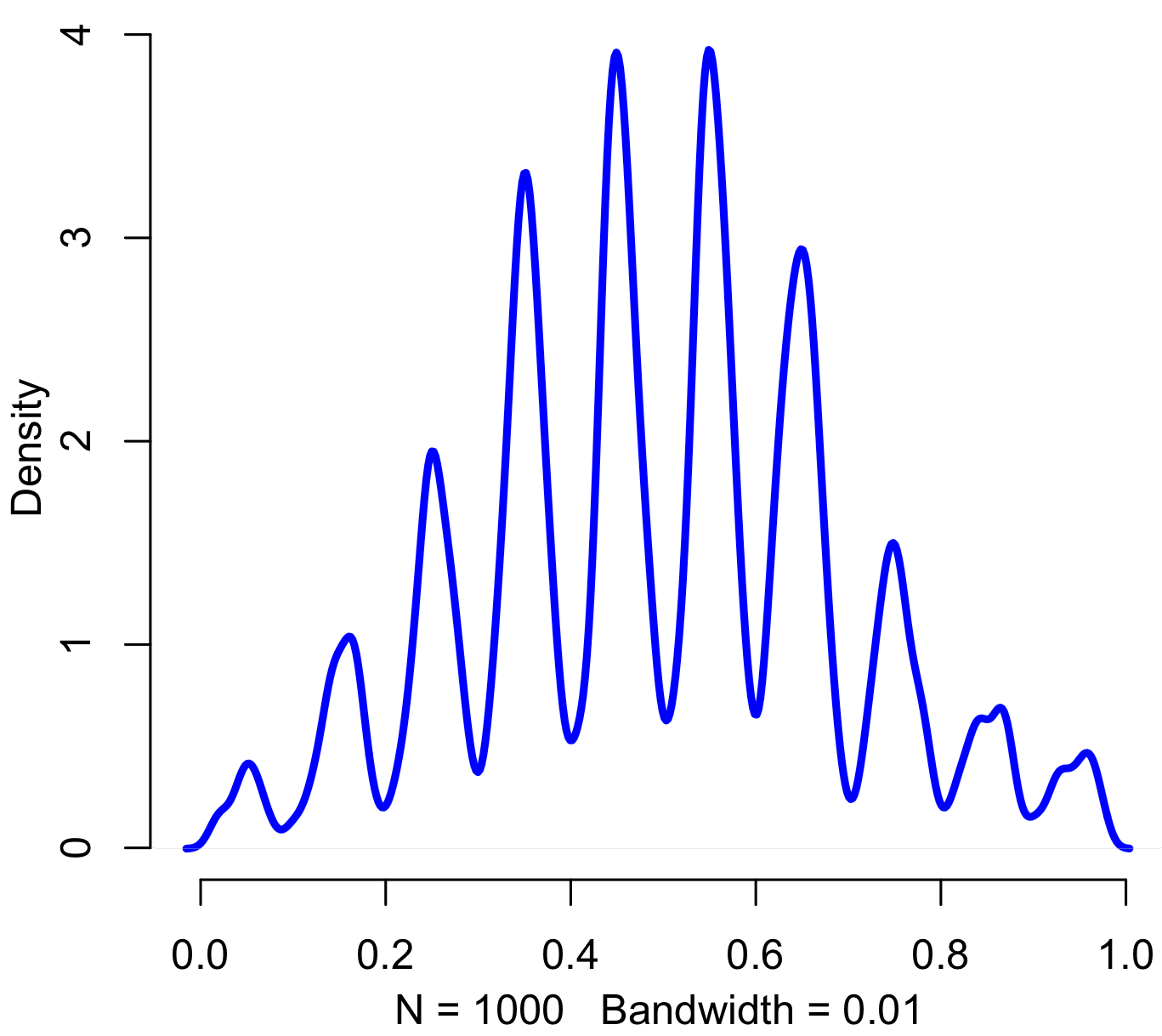[This article was first published on Probability and statistics blog » r, and kindly contributed to R-bloggers]. (You can report issue about the content on this page here)
Want to share your content on R-bloggers? click here if you have a blog, or here if you don't.Pinned, entropy augmented, digitally normal distribution, of no particular work-related use and thus perfectly suitable for today. Code in R:

```iters = 1000 sd = 2 precision = 20   results = rep(0,iters)   for(i in 1:iters) { x = floor(rnorm(20,5,sd) %% 10) results[i] = paste(c('.',x),sep="",collapse="") }   results = as.numeric(results)   plot(density(results,bw=.01),col="blue",lwd=3,bty="n")```